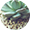《贵州省高等教育自学考试免考课程实施细则》
2019-01-311002018-10-101002018年贵州自考国家统考课程教材及大纲变更数据
2018-06-27100

\$(function(){ //导航 \$(".header .nav li a").eq(1).addClass("on"); initDeal(); }) var columnName = \$.trim(\$("#columnNameHidden").val()); // 初始链接处理 function initDeal(){ //var hostname = window.location.hostname; //hostname = hostname.split("."); var initHref = ""; // 页数判断 \$(".ggfy .num").each(function(i){ if(parseInt(\$.trim(\$(this).text())) === 1){ \$(this).attr("href","/gckh" + "/" + columnName); }else{ initHref = \$(this).attr("href"); \$(this).attr("href","/gckh" + "/" + columnName + "/" + initHref); } }); // 下一页判断 \$(".ggfy .sxy").each(function(i){ // 页数判断 if(\$.trim(\$(this).text()) === "下一页"){ // 判断是否需要跳转 if(\$.trim(\$(this).attr("href")).indexOf("page") == 0){ initHref = \$(this).attr("href"); \$(this).attr("href","/gckh" + "/" + columnName + "/" + initHref); } } }); } // 分页跳转 function turnPage(){ var pageNum = document.getElementById("turnPageNum").value; if(pageNum == null || pageNum == ""){ pageNum = 1; } if(!/^[1-9]\d*\$/.test(pageNum)){ pageNum = 1; } // 开始跳转 window.location = "/gckh" + "/" + columnName + "/" + "page" + pageNum + ".shtm"; }